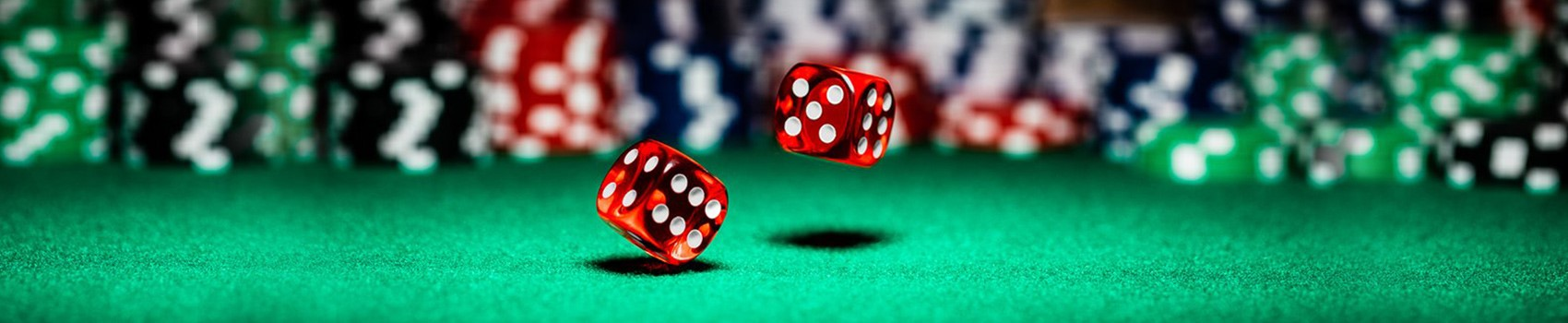# Golden Touch Craps

Can I improve my chances of winning with multiple bets?

A poster on the GTC private website had the following question:

My math mind is saying that the more bets you have on the board the better chance there is for a win. My gaming mind (experience) says this doesn't work. I think that the assumption that if you have two bets at 1.65 vig that the vig will go down to .83 and as far as I understand it does not.

I answered the posters question by giving an in depth explanation of the math involved when one makes multiple wagers. I am warning you in advance, this is strictly for those interested in numbers. If that is not "your thing" you are not going to enjoy this article.

Here is my answer to that poster:

Let me give you a specific example and see if that helps.

The house advantage (HA) on a place bet of the 6 or 8 is 1.52%. This means I can expect to lose 1.52% of All the money I wager when I place the 6 and/or 8 in a random game. If I just place the 6, I can expect to lose 1.52% of All the place bets I make on the 6. If I just place the 8, I can expect to lose 1.52% of All the place bets I make on the 8. If I place both the 6 and 8, I can expect to lose 1.52% of All the money I place on each of them.

That is the simple answer to your question. If you are curious and want to see how that works, I will now show how that calculation is made. If the math is confusing to you, then stop here and accept the above as being correct.

In a random game, I know that in the long run the probabilities of dice will average out and I can expect to see 5 sixes, 5 eights and 6 sevens thrown out of every 36 rolls. Of course it will not happen like this exactly every session or over a short number of rolls necessarily. But it will average out to that over a significantly large number of rolls.

Let us say I place the 6 only for \$6. On average over 36 rolls, I can expect to win \$7 on that bet 5 times and lose \$6 on it 6 times. Thus I can expect to win \$35 and lose \$36 for a net loss of \$1. I will have made this bet 11 times @ \$6 each, having wagered a total of \$66 on my place bet of the 6. My net loss (\$1) divided by my total amount wagered (\$66) yields a HA of 1/66 = 1.52%

Another way of looking at it is, I make a \$6 place bet on the 6, 11 times, wagering a total of \$66 on this place bet. I can expect to lose 1.52% of the \$66 I wagered on the 6 which equals 1.52% X \$66 = .0152 X \$66 = \$1

Obviously the same thing is true if I were to place the 8 instead because they both have the same probability of winning or losing.

Now let's look at what happens if I place the 6 and 8 together for \$6 each. I can expect to win my wager on the 6, 5 times out of 36. I can expect to win my wager on the 8, 5 times out of 36. I can expect to lose both bets 6 times out of 36.

This means I can expect to win \$7 on the 6, 5 times for \$35; I can expect to win \$7 on the 8, 5 times for \$35; I can expect to lose \$12 on the 6 and 8, 6 times for \$72. I will end up with \$70 - \$72 for a net loss of \$2. I will have wagered \$6 on the 6, the 5 times I win the bet for \$30, \$6 on the 8, the 5 times I win that bet for \$30 and \$12 on the 6 and 8, the 6 times I lose both bets for \$72. I will have wagered a total of \$30 + \$30 + \$72 = \$132 on the 6 and 8. Dividing my net loss (-\$2) by the total amount wagered (\$132) yields the following formula for the calculation of the HA. 2/132 = .0152 = 1.52%

I can also look at it as follows. If I wager a total of \$132 by placing both the 6 and 8, I can expect to lose 1.52% of the total amount wagered. 1.52% X \$132 = .0152 X \$132 = \$2

Let me know if this answers your question.